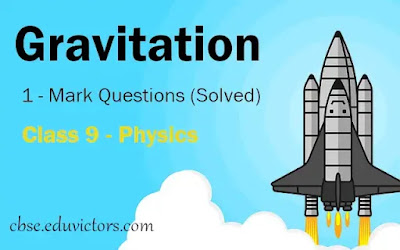# Gravitation 1 - Mark Questions (Solved) CBSE Class 9 - Physics

Q1: Who formulated the universal law of Gravitation?

Q2: What is free fall?

Answer: When an object falls from any height under the influence of gravitational force only, it is known as free fall.

Q3: What is the value of gravitational constant G on the earth? Does the value G changes on the moon?

Answer: Value of gravitational constant on the earth is 6.67×10⁻¹¹ Nm²/kg². Value of G does not change (universal constant), it remains the same on moon.

Q4: What will happen to gravitation force between two bodies, if mass of one body is doubled?

Answer: Gravitational force will double also.

Q5: Which force is responsible for the moon revolving round the earth?

Q6: How is gravity different from gravitation?

Answer: Gravitation is the force of attraction between any two bodies while gravity refers to attraction between any body and the earth.

Q7: What is acceleration due to gravity?

Answer: When an object falls towards the earth there is a change in its acceleration due to the gravitational force of the earth. So this acceleration is called acceleration due to gravity. It is denoted by symbol g.

Q8: Define weight of a body. What is the SI unit of weight?

Answer: The force with which the earth attracts a body towards its centre is called weight. The SI unit of weight is Newton (N). W = mg

Q9: Is value of g same on the earth and the moon?

Answer: Value of g varies planet to planet. It has different values for the earth and the moon.

Q10: Why is value of 'g; slightly higher at poles (on the earth) than that of at the equator?

Answer:   As the radius of the earth increases from the poles to the equator, the value of g becomes greater at the poles than at the equator.

Q11: As we go higher (at large heights), what happens to the value of g?

Answer: As we go at large heights, value of g decreases.

Q12: Name the scientist who gave the three laws of planetary motion.

Q13: Which Kepler's law led Newton to establish inverse square rule in law of gravitation?

Answer: Kepler's Third law i.e. T²  ∝ r³

Q14: What type of force is involved in the formation of tides in the sea?

Answer: Gravitation force acted by the moon on the earth.

Q15: What is the weight of a 1 kilogram mass on the earth? (g = 9.8 m/s²).

Answer: W = mg = 1kg × 9.8m/s² = 9.8 N

Q16:  Is weight a force?

Q17: When a body is dropped from a height, what is its initial velocity?

Q18: On what factor/factors does the weight of a body depend?

Answer: Since W = mg. It depends on
(i) mass of the object
(ii) value of 'g' on that planet.

Q19: A body has a weight of 10 kg on the surface of earth. What will be its weight when taken to the centre of the earth?

Answer: At the centre of the earth value of g = 0 m/s². Thus, weight = 0N

Q20: The earth is acted upon the gravitation of the sun, even though it does not fall into the sun. Why?

Answer: The gravitational force is responsible for providing the necessary centrepetal force. It allows the earth to revolve around the sun in defined circular orbit.

Q21: The earth attracts the apple. Does the apple attract the earth also?

Q22: Can gravitational force be repulsive?

Answer: Never. It is always an attractive force.

Q23: If the weight of a body on the earth is 6 N, what will it be on the moon?

Answer: If the weight of a body on the earth is 6N, then its weight on the moon would be about 1N. This is because acceleration due to gravity on the surface of the moon is 1/6th the acceleration due to gravity on the earth’s surface.

Q24: Is Newton's third law applicable to the gravitational force?

Q25: If a stone is thrown upwards, while calculating the maximum height it reaches, acceleration due to gravity is taken as positive or negative value?

Q26: Which is more fundamental, the mass of a body or its weight? Why?

Answer: Mass is more fundamental because it remains constant at all places, irrespective of a change in place. But weight depends on acceleration due to gravity g, which varies with location.

Q27: Two stones of mass 1kg and 10kg are dropped simultaneously from the top of a tower of 100m. Assuming negligible air resistance, which stone will react the ground first?

Answer: Both will reach ground at the  same time.

Q28: Why one can jump higher on the surface of moon than on the earth ?

Answer: Because the value of acceleration due to gravity (g) at moon surface is nearly 1/6th of that at the surface of earth.

Q29: What kind of quantity is weight – a scalar or a vector?

Answer: Weight is a vector quantity.

Q30: Who found out the value of gravitational constant (G)?